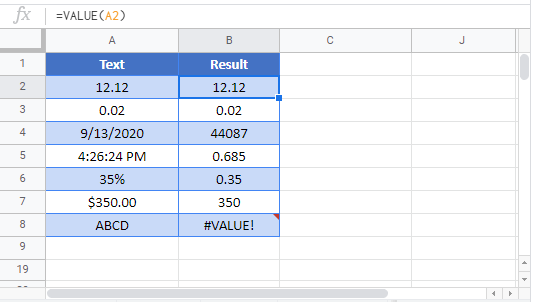# VALUE Function – Text to Number – Excel & Google Sheets

This Tutorial demonstrates how to use the Excel VALUE Function in Excel to convert a number stored as text into a number.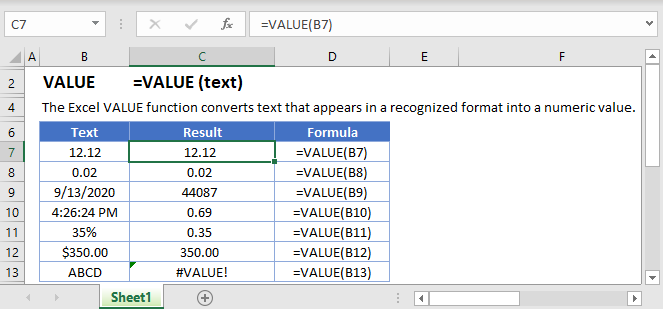## VALUE Function Description:

The VALUE Function Converts a number stored as text into a number.

To use the VALUE Excel Worksheet Function, select a cell and type: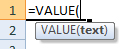(Notice how the formula inputs appear)

## VALUE function Syntax and inputs:

`=VALUE(text)`

text – A string of text. Reference a cell containing text, reference a cell whose formula results in text, or enter text directly into the Value function using parenthesis (example: “string of text”).

## How to use the VALUE Function in Excel:

The VALUE function converts a text string that represents a number into a number. The underlined portion is important because it can’t convert “abc” (not a number) into a number.

`=VALUE(B2)`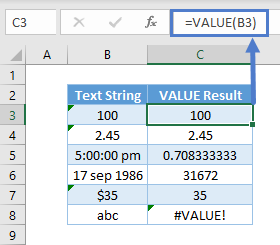As seen above; it could be a whole number, decimal, time (which is stored as a decimal), date (which is stored as a whole number), or numbers with currency. It doesn’t work only for the last item, as “abs” is not a number.

## Convert Text Results into Numbers

You might use Text formulas such as CONCATENATE, TRIM, LEFT, MID, RIGHT, etc to produce a result that represents a number. You need to use VALUE to convert it into a number. Otherwise, you cannot sum them up or use it properly in filters and PivotTables.

Without VALUE, you see cell F3 unable to sum the numbers up.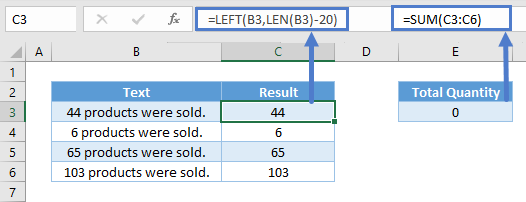And here’s the formula with VALUE.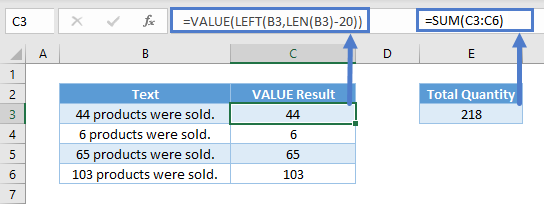## VALUE Function in Google Sheets

The VALUE Function works exactly the same in Google Sheets as in Excel: